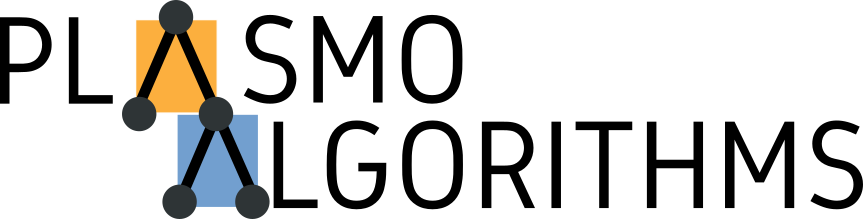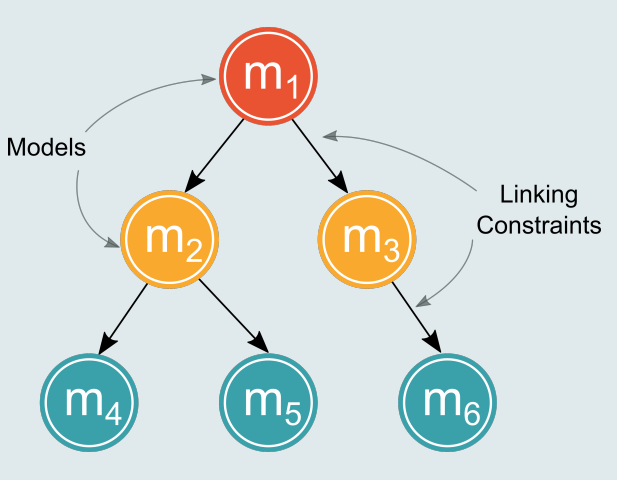dummy-link

# PlasmoAlgorithms

9

1

9

4

### contributors

#### ReadmePlasmoAlgorithms is a collection of decomposition algorithms to solve mathematical programming models taking Plasmo graphs as input. Plasmo graphs allow to create graphs of models, in which each node represents a model, and the arcs represent linking constraints. Several hierarchical problems can be expressed in this way, for example: supply chain planning and scheduling, optimization of power systems, and stochastic programming problems.The algorithms implemented are:

• Lagrange Decomposition
• Multilevel Benders Decomposition

## Installation

``````(v1.0) pkg> add https://github.com/jalving/Plasmo.jl.git
(v1.0) pkg> add https://github.com/bbrunaud/PlasmoAlgorithms.jl.git
``````

## Usage

### Generate the graph

``````using JuMP
using Gurobi
using Plasmo
using PlasmoAlgorithms

## Model on x
# Min 16x + 10x
# s.t. x + x <= 1OutputFlag=0
#      x ∈ {0,1}
m1 = Model(solver=GurobiSolver(OutputFlag=0))
@variable(m1, xm[i in 1:2],Bin)
@constraint(m1, xm + xm <= 1)
@objective(m1, Max, 16xm + 10xm)

## Model on y`
# Max  4y
# s.t. y + y <= 1
#      8x + 2x + y + 4y <= 10
#      x, y ∈ {0,1}
m2 = Model(solver=GurobiSolver(OutputFlag=0))
@variable(m2, xs[i in 1:2],Bin)
@variable(m2, y[i in 1:2], Bin)
@constraint(m2, y + y <= 1)
@constraint(m2, 8xs + 2xs + y + 4y <= 10)
@objective(m2, Max, 4y)

## Plasmo Graph
g = ModelGraph()
g.solver = GurobiSolver(OutputFlag=0)
n1 = add_node(g)
setmodel(n1,m1)
n2 = add_node(g)
setmodel(n2,m2)

## Linking
@linkconstraint(g, [i in 1:2], n1[:xm][i] == n2[:xs][i])
```

### To solve with Lagrange
``````

solution = lagrangesolve(g,options...)

``````
### To solve with Benders
``````

solution = bendersolve(g,options...)

``````The algorithms will return a solution object with all relevant information about the solution process

## Lagrange Decomposition
The Lagrangean decomposition algorithm will dualize all linking constraints for any arbitrary graph. It could be a tree, it could be a sequence of nodes connected (e.g. temporal decomposition), or it may even contain cycles.

### Function documentation
`lagrangesolve(g::PlasmoGraph;update_method,ϵ,timelimit,lagrangeheuristic,initialmultipliers,,α,δ,maxnoimprove,cpbound)`, solves the input graph using the lagrangean decomposition algorithm

### Options

* `update_method` Multiplier update method
* allowed values: `:subgradient, :probingsubgradient, :marchingstep, :intersectionstep, :cuttingplanes`
* default: `:subgradient`
* `ϵ` Convergence tolerance
- default: 0.001
* `timelimit` Algorithm time limit in seconds
- default: 3600 (1 hour)
* `lagrangeheuristic` Function to solve the lagrangean heuristic. PlasmoAlgorithms provides 2 heuristic functions: `fixbinaries, fixintegers`
- default: `fixbinaries`
* `initialmultipliers` initialization method for lagrangean multipliers. When `:relaxation` is selected the algorithm will use the multipliers from the LP relaxation
- allowed values: `:zero,:relaxation`
- default: `zero`
* `α` Initial value for the step parameter in subgradient methods
- default: 2
* `δ` Shrinking factor for `α`
- default: 0.5
* `maxnoimprove` Number of iterations without improvement before shrinking `α`
- default: 3

### Multiplier updated methods
It supports the following methods for updating the lagrangean multipliers:
* Subgradient
* Probing Subgradient
* Marching Step
* Intersection Step (experimental)
* Interactive
* Cutting Planes
* Cutting planes with trust region
* Levels
``````

08/07/2017

#### Last Touched

about 2 months ago

175 commits